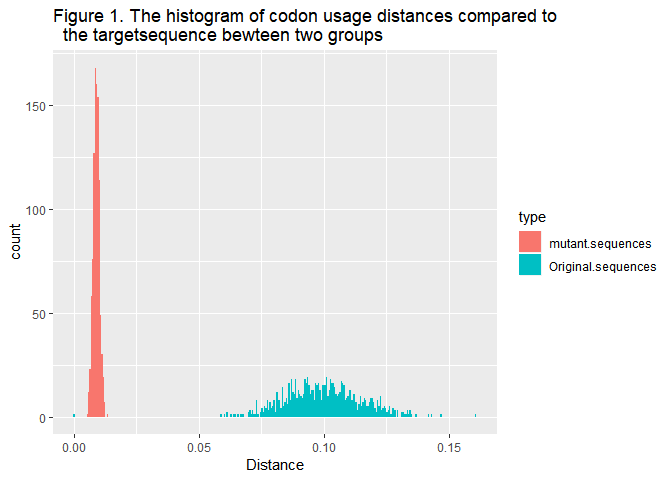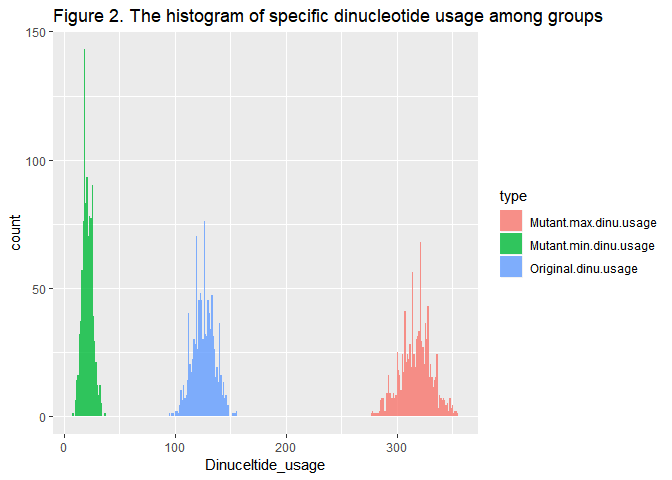# Strategies for codon_mimic and dinu_to

The implementation of the mutation functions in the SynMut package were briefly described in the help documents of the functions, which can be accessed by ?codon_random or help("codon_random"). In this part I will introduce our logic behind the optimization problems in codon_mimic and dinu_to.

### Function codon_mimic

The codon usage bias generally refers to the difference in the relative usage of synonymous codons. To mimic a target codon usage bias equals to minimizing the difference of the relative synonymous codon frequency between the current and target DNA sequences.

Codon usage mimicking is straightforward when the whole sequence is mutable: for all the synonymous codons in the whole sequence, we re-arrange their relative weights to the target. For example, There is a total of 100 codons (AAA or AAG) coding for Lysine in the original sequence, and the relative frequency of these synonymous codons in the target sequence is AAA : AAG = 1 : 3, then the desired number of codon AAA and AAG in the mutant sequence would be 25 and 75 respectively. These 25 AAA and 75 AAG codons will be randomly assigned back to their original codon positions. This process will iterate through all the synonymous codon sets, and finally forming a mutant sequence mimicking the target codon usage.

However if there are fixed (not mutable) regions in the original sequence which prevent mutations, similar to codon_to with regions, the first step is to extract the mutable regions. In this scenario, we have to adjust for the fixed codons while calculating the desired number of different synonymous codons in the mutable regions. Sometimes we may find the number of a specific codon in the fixed region is already greater than what we expect it to be, in this case we will leave this codon and only mimic the distribution of the rest synonyms. The ideal design of codon_mimic is not unique as it allows swaps between positions of the synonymous codons.

We evalutaed the goodness of the mutation by calculating the codon usage distance (by least squares approach) between the original and the codon_mimic mutated sequences, compared to the distances between the original and the randomly mutated sequences.

library(SynMut)
# Generate 1000 random DNA seuqneces of length 2019nt.
set.seed(2019)
ori.seq <- seq_random(n = 1000, m = 2019)

# Randomly select one sequence as the target sequnece to represent the target
# codon usage. Calculate the codon usage distance betwee all the sequences and
# the target.
target.seq <- ori.seq[sample(1:1000,1)]
seq.dist <- mapply(function(x, y){
codon_dist(Biostrings::DNAStringSet(x), y)
}, ori.seq, list(target.seq))

# Generate codon_mimic mutant sequences basing on the previous-generated random
# sequences, mimicing the target codon usage, and calculate the codon usage
# distance.
mut.mimic.dist <- sapply(ori.seq, function(x){
mut.seq.tmp <- codon_mimic(Biostrings::DNAStringSet(x), target.seq)
codon_dist(mut.seq.tmp, target.seq)
})

# Plot the distribution
library(ggplot2)
df <- data.frame(Original.sequences = seq.dist, mutant.sequences = mut.mimic.dist)
df <- tidyr::gather(df, "type", "Distance", 1:2)
ggplot(df, aes(x = Distance, y = ..count.., fill = type, group = type)) +
geom_histogram(position = "identity", bins = 300) +
ggtitle("Figure 1. The histogram of codon usage distances compared to
the targetsequence bewteen two groups")As shown in Figure 1, the codon usage distances of the mutants were well clusetered within a narrow range with a significantly smaller mean, indicating that the results from codon_mimic function are stable and significant.

### Function dinu_to

The dinu_to function allows maximizing or minimizing certain dinucleotide content in the input DNA sequences, either preserving or allowing altering the original codon usage bias. This function was implemented with a heuristic greedy approach.

Dinucleotides can be characterized to three categorizes according to their positions in the codons. Dinucleotide12 are the dinucleotides formed by the first two mononucleotides at a codon, dinucleotide23 consist the latter two bases of the codon, and dinucleotide31 are the dinucleotides at the codon-codon junctions.

The dinu_to problem is essential an optimization problem, and it would be hard to find a best result. Our heuristic greedy solution can provide a good but not neccessarily the best result. The strategy includes three main steps:

1. To determine the “optimal codon” in each synonymous codon set. To retain/remove as much target dinucleotide as posiible, we have to identify the “optimal codon” for mutation. The “optimal codon” was filtered in order by ther follwing criteria: 1. the codon itself includes/not includes the target dinucleotide in position12 or position23. 2. the first base and the third base of the codon should have/not have the potential for forming a target dinucleotide in terms of dinucletide31. If there are multiple equivalent choices after filtering, we will pick a random one.
2. To mutate all the synonymous codons to their correspondant optimal codon. The second step is to mutate the codons in the original sequence to their synonymous optimal substitude we identified in the previous step.
3. Swaps between the synonymous codons to build/destruct dinucleotide31. The last step is to swap the positions of the synonymous codons in the sequences, in order to build/destruct the target dinucleotide in position31. This process will not affect the codon usage, however it will change the dinucleotide composition via altering the composition of dinucleotide31.

The keep = True argument of the function ensures mutations without changing the codon usage in the original sequence. It was achieved by only performing the step 3 , which will only affect the distribution of dinucleotide31. The default keep = False allows mutations that can change the codon usage pattern, and also more extreme dinucleotide usage.

# Generate 1000 random DNA seuqneces of length 2019nt.
set.seed(2019)
ori.seq <- seq_random(n = 1000, m = 2019)

# Randomly select one dinucleotide as the target dinucleotide for this example.
target.dinu <- sample(seqinr::words(2), 1)
target.dinu
##  "ta"
dinu.index <- which(seqinr::words(2) == target.dinu)
# Capture the dinuceltide usage in the sequences
seq.dinu <- sapply(ori.seq, function(x){
get_du(Biostrings::DNAStringSet(x))[,dinu.index]
})

# Generate dinu_to mutant sequences basing on the previous-generated random
# sequences, maximizing and minimizing the target dinucleotide, and calculate
# the frequency of target dinucleotide in the mutant sequences.
mut.max.dinu <- sapply(ori.seq, function(x){
seq.tmp <- input_seq(Biostrings::DNAStringSet(x))
mut.seq.tmp <- dinu_to(seq.tmp, max.dinu = target.dinu)
get_du(mut.seq.tmp)[,dinu.index]
})
mut.min.dinu <- sapply(ori.seq, function(x){
seq.tmp <- input_seq(Biostrings::DNAStringSet(x))
mut.seq.tmp <- dinu_to(seq.tmp, min.dinu = target.dinu)
get_du(mut.seq.tmp)[,dinu.index]
})

# Plot the distribution
df <- data.frame(Original.dinu.usage = seq.dinu, Mutant.max.dinu.usage = mut.max.dinu,
Mutant.min.dinu.usage = mut.min.dinu)
df <- tidyr::gather(df, "type", "Dinuceltide_usage", 1:3)
ggplot(df, aes(x = Dinuceltide_usage, y = ..count.., fill = type, group = type)) +
geom_histogram(position = "identity", bins = 300, alpha = 0.8) +
ggtitle("Figure 2. The histogram of specific dinucleotide usage among groups")In the Figure 2 above, the usage of the target dinuceltide was significantly different among three groups, where the dinucleotide-minimized mutant sequences having the lowest usage and the dinucleotide-maximized sequneces having the highest usage, and the original sequences at the middle.